# KCET 2016 Chemistry Paper with Solutions

KCET 2016 solved question paper will help the students to prepare efficiently for the KCET exams by understanding the distribution of marks for different topics across the subjects. Practising KCET previous year question papers with answer keys will help the candidates to face the exam with more confidence. This set of solutions is designed to help the aspirants in achieving time management skills and also acts as an effective aid in their preparation.

### KCET 2016 - Chemistry

Question 1: The half-life period of a 1st order reaction is 60 minutes. What percentage will be left over after 240 minutes?

1. a. 6.25 %
2. b. 1.25%
3. c. 5%
4. d. 6 %

Solution:

Given that:

Half-life (t1/2) = 60 min.

Percentage left after 240 min = ?

From Ist order kinetic equation t1/2 = (2.303 / k) log {a / (a - [a / 2])}

t1/2 = (2.303 / k) log 2

k = [2.303 / 60] log 2 min-1

Again from Ist order kinetic equation t = (2.303 / k) log (a / [a - x])

log (a / [a - x]) = [t . k] / [2.303]

= [240 min × 2.303 × log 2] / [60 × 2.303]

log (a / [a - x]) = 4log 2

(a / [a - x]) = 24

(a / [a - x]) = 16

If initial concentration (a) taken as 100%

Then, % of the reactant left after 240 min = 100 / [a - x] = 16

[a - x] = [100 / 16] %

[a - x] = 6.25%

Question 2: Which of the following is not a colligative property?

1. a. Osmotic pressure
2. b. Optical activity
3. c. Depression
4. d. Elevation in Boiling point

Solution:

Colligative properties of solutions are properties that depend upon the concentration of solute molecules or ions, but not upon the identity of the solute. Colligative properties include vapour pressure lowering, boiling point elevation, freezing point depression and osmotic pressure. Whereas optical activity is the ability of a chiral molecule to rotate the plane of plane-polarized light. So, optical activity is not a colligative property.

Question 3: The contribution of the particle at the edge centre to a particular unit cell is,

1. a. 1 / 2
2. b. 1 / 4
3. c. 1
4. d. 1 / 8

Solution:

An atom present at the edge centre of a unit cell is shared by four-unit cells.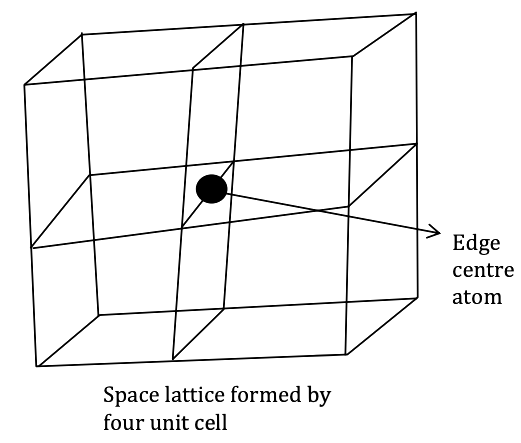The contribution from each edge centre atom is thus ¼.

Question 4: When an electrolyte is dissociated in solution, the van’t Hoff’s factor (i) is,

1. a. > 1
2. b. < 1
3. c. = 0
4. d. = 1

Solution:

Van’t Hoff factor is the ratio of the concentration of particles formed when a substance is dissolved to the concentration of the substance by mass.

i = measured value / Calculated value

Electrolytes are dissociated in the solutions, for dissociation in absence of association, the van’t Hoff factor is i > 1.

Question 5: Which of the following is incorrect in a galvanic cell?

1. a. Oxidation occurs at anode
2. b. Reduction occurs at cathode
3. c. The electrode at which electrons are gained is called the cathode.
4. d. The electrode at which electrons are lost is called the cathode.

Solution:

Galvanic Cell also called as voltaic Cell. In Galvanic Cell at Cathode reduction will take place (gain of e– will take place). So it acts as a sink of electrons. On Cathode positive polarity developed. The anode is of negative polarity. On anode, oxidation will take place. So it acts as a source of electrons.

Question 6: A secondary cell is one

1. a. can be recharged
2. b. can be recharged by passing a current through it in the same direction
3. c. can be recharged by passing a current through it in the opposite direction
4. d. cannot be recharged

Solution:

A Secondary Cell is a type of electrical battery. In secondary Cells, the reaction can be reversed by passing a current through it in the opposite direction. That is why these cells can be recharged by passing electric current and can be used again and again. These Cells also called storage Cells.

Question 7: Osmotic pressure of the solution can be increased by,

1. a. increasing the temperature of the solution
2. b. decreasing the temperature of the solution
3. c. increasing the volume of the vessel
4. d. diluting the solution

Solution:

Osmotic pressure (π) of a solution is affected by the concentration of solute and temperature.

As we know π = CRT

Here π is as given below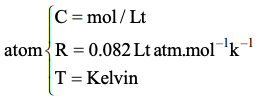So, π ∝ Concentration (molarity)

π ∝ T

Thus osmotic pressure is directly proportion to temperature. So Osmotic pressure increased with the increase in temperature.

Question 8: The amount of current in Faraday is required for the reduction of 1 mol of Cr2O7-- ions to Cr3+ is,

1. a. 1F
2. b. 2F
3. c. 6F
4. d. 4F

Solution:

Cr+62O7 + 6e- → Cr+3

Oxidation state of chromium consider as x,

2x + 7 (–2) = –2

x = [14 - 2] / 2 = +6

Electrons required for reduction of two chromium ion will be:

2 (6 – 3) = 6e

Charge required for 1 mole e = 1F Charge

So, Charge required for 6-mole e = 6F Charge

Question 9: For a chemical reaction, mA → xB, the rate law is r = k[A]2. If the concentration of A is doubled, the reaction rate will be,

1. a. Doubled
3. c. Increases by 8 times
4. d. Unchanged

Solution:

From a chemical reaction mA → xB

The rate law (r) = k [A]2

When the concentration is doubled, then r = k 2

r = 4k

The rate of reaction will be quadrupled.

Question 10: Schottky defect in a crystal is observed when,

1. a. Unequal number of cations and anions are missing from the lattice.
2. b. Equal number of cations and anions are missing from the lattice.
3. c. An ion leaves its normal site and occupies an interstitial site.
4. d. No ion is missing from its lattice site

Solution:

Schottky defect is a type of point defect or imperfection in solid arises when an equal number of cations and anions are missing from the lattice. Where both Cations and anions are of the same size.

Question 11: 3A → 2B rate of reaction + d[B] / dt is equal to

1. a. (-3 / 2) (d[A] / dt)
2. b. (-2 / 3) (d[A] / dt)
3. c. (+2) (d[A] / dt)
4. d. (-1 / 3) (d[A] / dt)

Solution:

For the given reaction: 3A → 2B

Rate of disappearance of A = (-1 / 3) (d[A] / dt)

Rate of appearance of B = +(1 / 2) (d[B] / dt)

So, the overall rate of the reaction is (-1 / 3) (d[A] / dt) = +(1 / 2) (d[B] / dt)

(-2 / 3) (d[A] / dt)

Question 12: The activation energy of a chemical reaction can be determined by,

1. a. evaluating rate constants at two different temperatures
2. b. changing the concentration of reactants
3. c. evaluating the concentration of reactants at two different temperatures
4. d. evaluating rate constant at standard temperature

Solution:

From the Arrhenius equation: K = Ae-Ea/RT is the rate constant

Ea = activation energy

R = gas constant

T= Temperature (in Kelvin)

A = Arrhenius factor

The activation energy can also be calculated directly given two known temperatures and a rate constant at each temperature. Suppose that at two different temperatures T1 and T2, reaction rate constants k1 and k2.

ln (k1 / k2) = [(1 / T2) - (1 / T1)] (Ea / R) - Arrhenius equation

Question 13: Which of the following statements is incorrect w.r.t. Physisorption?

1. a. The forces involved are van der Waal’s forces.
2. b. More easily liquefiable gases are adsorbed easily
3. c. Under high pressure, it results into Multi-molecular layer on the adsorbent surface.
4. d. ΔAdsorption is low and +Ve

Solution:

Physisorption: Adsorption is caused by van der waals forces. It is an exothermic process.

The enthalpy change for physical adsorption: -

ΔH = ΔG + TΔS

The entropy change of the adsorbate S necessarily negative since the adsorbed state is more ordered than the unadsorbed state due to a loss of at least one degree of freedom.

The ΔG is negative for spontaneity of the adsorption process. Hence, enthalpy change H accompanying physical adsorption is always negative (exothermic).

Question 14: Sulphur sol contains

1. a. Discrete S-atoms
2. b. Discrete S-molecules
3. c. Large aggregates of S-molecules
4. d. Water dispersed in Solid Sulphur

Solution:

Sulphur sols are colloidal solutions of elemental Sulphur or of Sulphur rich compounds. The particle in this solution have diameters of 0.1 – 1.0 µm and consist large aggregation of S8 molecules (Hydrophobic sols) or of chain-like Sulphur compounds with Hydrophilic end.

Question 15: Reactions in Zeolite catalyst depend on,

1. a. Pores
2. b. Apertures
3. c. Size of cavity
4. d. All of these

Solution:

The Characteristic feature of Zeolites is the openness of the structures. Which permit cavities of different sizes.

The reaction – selectivity of Zeolites depends upon the size of cavities (Cages), pores (apertures) and the distribution of pores in the structure. The pore size in Zeolites generally varies from 260 pm to 740 pm.

Question 16: IUPAC name of the compound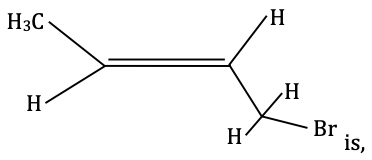1. a. 1-Bromo but-2-ene
2. b. 2-Bromo-2-butene
3. c. Bromo butene
4. d. 1-Bromo but-3-ene

Solution: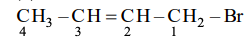First, select the longest carbon chain (parent chain). Then, parent chain numbed so that the multiple bond and substituent have the lowest number. For double bond ‘ene’ suffix is used.

IUPAC name of this compound: 1–bromobut–2–ene.

Question 17: Replacement of Cl of Chlorobenzene to give phenol requires drastic conditions, but Cl of 2, 4-dinitro chlorobenzene is readily replaced. This is because

1. a. –NO2 group makes the ring electron-rich at ortho and para positions
2. b. –NO2 group withdraws electrons from meta position
3. c. –NO2 donate electrons at meta position
4. d. –NO2 withdraws electrons from ortho and para positions

Solution:

Replacement of Cl group by OH group is a nucleophilic substitution reaction.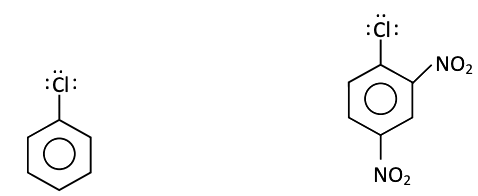In Chlorobenzene, lone pair of chlorine involves in resonance and develop partial double bond character, the bond gets stronger. Hence, requires drastic conditions to form phenol.

But in 2, 4–dinitrochlorobenzene, –NO2 group is an electron-withdrawing group and deactivates ortho and para position as compared to meta position. Which decrease the density of benzene ring. So, Chlorine can be easily replaced at normal condition.

Question 18: In the reaction :

Ethanolthe product Z is,

1. a. C2H4
2. b. CH3CH2OCH2CH3
3. c. CH3CH2OSO3H
4. d.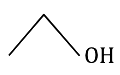Solution: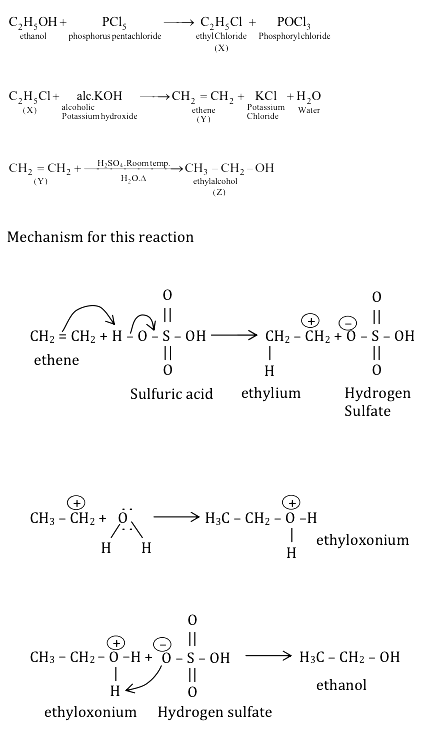Question 19: Which of the following compound is most acidic?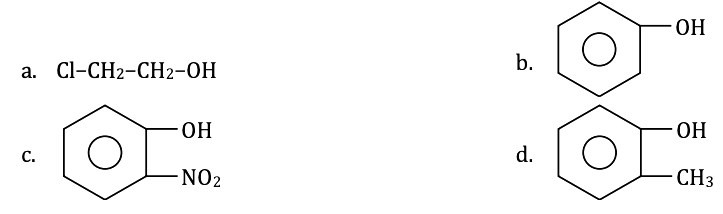Solution:

The Nitro group is an electron-withdrawing in nature. In o–nitrobenzene after removal of the Hydrogen atom, the structure is resonance stabilizes and being more acidic than other compounds.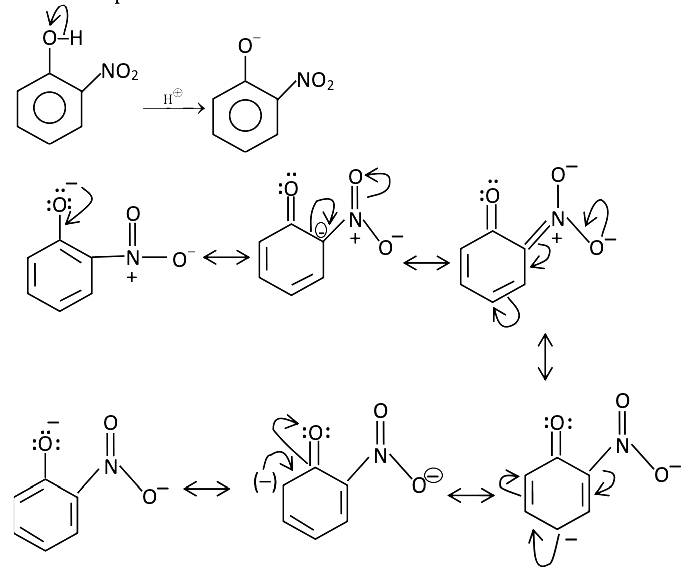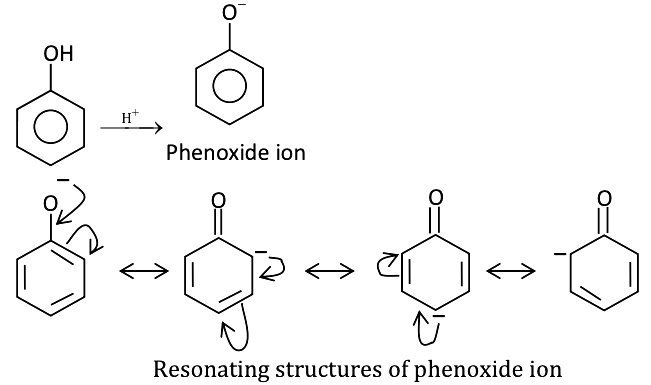The number of resonating structure decides the stability of compounds. Phenol is more stable than alcohol and less acidic than o-nitro phenol. m-cresol, CH3 group is e donating in nature so removal of H+ ion is less favourable as compared to another compound.

Question 20: Benzene carbaldehyde is reacted with concentrated NaOH solution to give the products A and B. the product A can be used as a food preservative and the product B is an aromatic hydroxyl compound where OH group is linked to sp3 hybridised carbon atom next to Benzene ring. The products A and B are respectively,

1. a. Sodium benzoate and phenol
2. b. Sodium benzoate and phenyl methanol
3. c. Sodium benzoate and cresol
4. d. Sodium benzoate and picric acid

Solution:

Benzaldehyde or an aldehyde without active hydrogen undergoes a redox reaction under the action of a strong base, known as Cannizzaro reaction. It undergoes disproportionation reaction under the action of a strong base (NaOH) to form a molecule of alcohol and carboxylic acid or sodium benzoate which can be used as a food preservative to extend shelf life. The chemical equation is: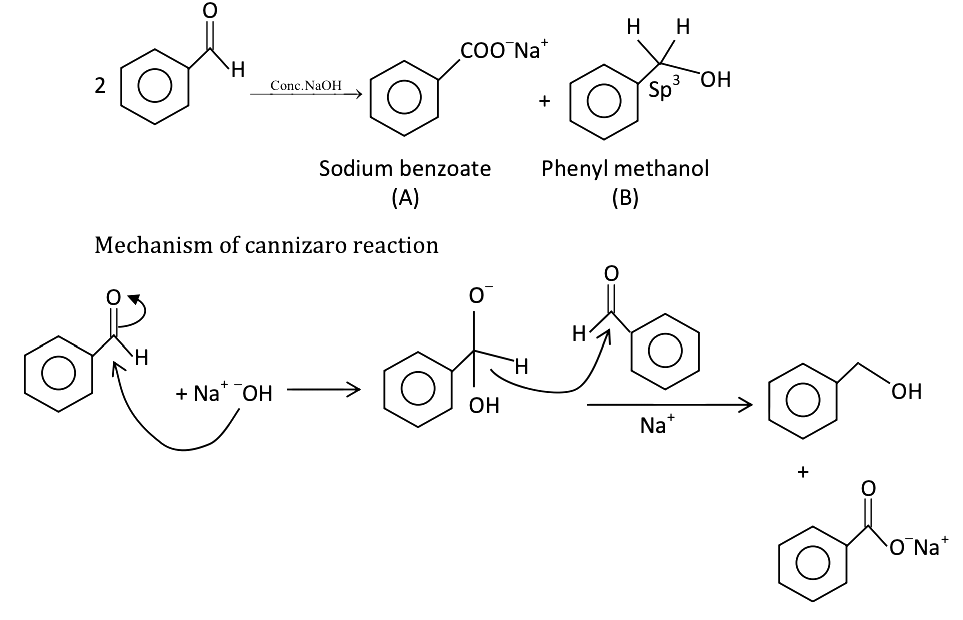Question 21: The reaction which involves dichlorocarbene as an electrophile is,

1. a. Reimer-Tiemann reaction
2. b. Kolbe’s reaction
3. c. Friedel-Craft’s acylation
4. d. Fittig’s reaction

Solution:

Reimer – Tiemann reaction

This reaction used to convert phenol to an o-hydroxy benzaldehyde using chloroform, a base and acid workup.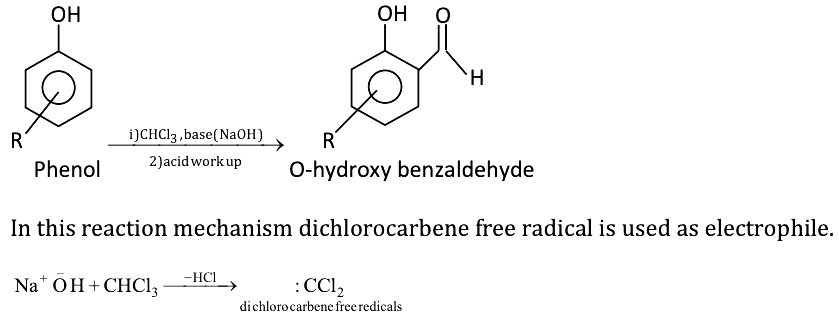Question 22: Ethanol is converted into ethoxy ethane,

1. a. By heating excess of ethanol with conc. H2SO4 at 140 °C.
2. b. By heating Ethanol with an excess of conc. H2SO4 at 443 K.
3. c. By treating with conc. H2SO4 at room temperature.
4. d. By treating with conc. H2SO4 at 273 K.

Solution: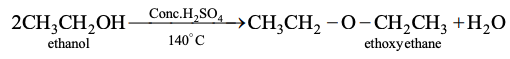Due to the presence of lone pairs of the electron in oxygen, ethanol acts as a weak base, it reacts with strong mineral acids.

Convention of ethanol to ethoxy ethane follows SN2 mechanism.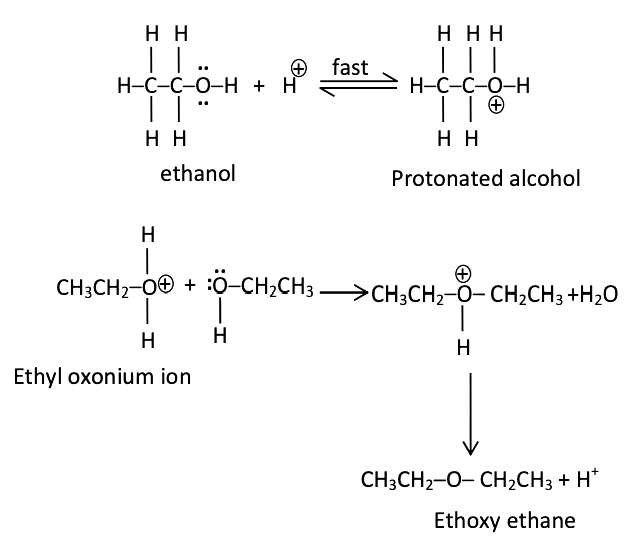Question 23: An organic compound X is oxidized by using an acidified K2Cr2O7 solution. The product obtained reacts with phenylhydrazine but does not answer the silver mirror test. The compound X is,

1. a. 2-propanol
2. b. Ethanal
3. c. Ethanol
4. d. CH3CH2CH3

Solution:

On oxidation of compound (x) in presence of strong oxidizing agent oxidation product is formed, this oxidation production reacts with phenylhydrazine that means it is a carbonyl group (aldehyde or ketone) containing compound.

Silver mirror test only is given by aldehydes, not by Ketones. Thus product must be ketone.

As we know primary alcohol oxidized to give aldehyde whenever secondary alcohol oxidised to give ketone.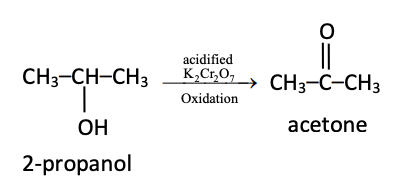Question 24: Predict the product ‘C’ in the following series of reactions: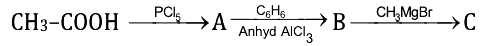1. a.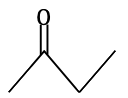2. b. CH3CH(OH)C6H5
3. c. CH3CH(OH)C2H5
4. d. (CH3)2C(OH)C6H5

Solution: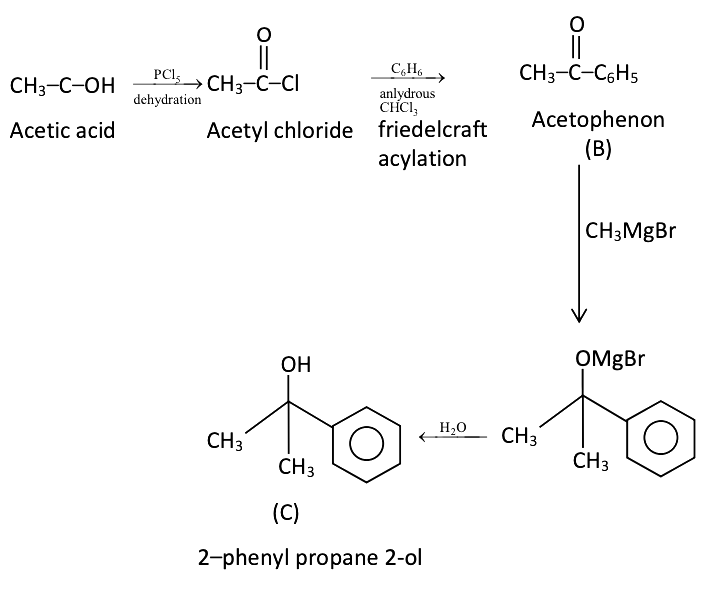Question 25: The number of oxygen atoms in 4.4 gm of CO2 is,

1. a. 1.2 × 1023
2. b. 6 × 1022
3. c. 6 × 1023
4. d. 12 × 1023

Solution:

Molar mass of CO2 (Mwt) = 44 g/mol

Given the mass of CO2 (w) = 4.4 g

Moles of CO2 (n) = given mass / molecular mass = 4.4 / 44 = 0.1mol

One CO2 molecule having two oxygen atoms

So, the total number of oxygen – atom = 2 × number of moles × NA

= 2 × 0.1 × 6.023 × 1023 atoms

= 1.2 × 1023 atoms

Question 26: If the bond energies of H–H, Br–Br H–Br are 433, 192 and 364 kJ mol–1 respectively, then ΔH° for the reaction: H2(g) + Br2(g) → 2HBr(g) is

1. a. – 261 kJ
2. b. + 103 kJ
3. c. +261 kJ
4. d. –103 kJ

Solution:

Given that:

Bond energies of H–H = 433 KJ mol-1

Bond energies of Br–Br = 192 KJ mol-1

Bond energies of H–Br = 364 KJ mol-1

H – H + Br – Br → 2H – Br

ΔH° = ∑ bond energy (reactant) – ∑ bond energy (products)

= 433 + 192 – 2 × 364

= +625 – 728

= – 103 KJ

Question 27: In the reaction ; Fe(OH)3(s) ⇌ Fe3+(eq) + 3OH(aq), if the concentration of OH ions is decreased by 1 / 4 times, then the equilibrium concentration of Fe3+ will increase by,

1. a. 8 times
2. b. 16 times
3. c. 64 times
4. d. 4 times

Solution:

For the reaction:

Fe(OH)3(s) ⇌ Fe3+(eq) + 3OH(aq)

Equilibrium constant (k) = [Fe+3] [-OH]3

If the concentration of OH ion is decreased by ¼ times,

Now, K = [Fe+3] [-OH / 4]3

K = [Fe+3] [-OH / 64]

So, at equilibrium concentration of Fe+3 is increased by 64 times.

So that equilibrium constant does not change.

Question 28: The correct statement regarding entropy is,

1. a. At absolute zero temperature, the entropy of a perfectly crystalline solid is zero.
2. b. At absolute zero temperature, the entropy of a perfectly crystalline substance is +Ve.
3. c. At absolute zero temperature, the entropy of all crystalline substances is zero.
4. d. At 0 °C, the entropy of a perfect crystalline solid is zero.

Solution:

The third law of thermodynamics tells us that all molecular movement stops at a temperature we call absolute zero or zero (0) Kelvin (-273oC)

Since temperature is a measure of molecule movement, there can be no temperature lower than absolute zero. At this temp., a perfect crystal has no disorder.

The entropy (s) of a pure perfectly crystalline compound at T = 0K is zero (no disorder).

ST=0 = 0

Question 29: Equilibrium constants K1 and K2 for the following equilibrium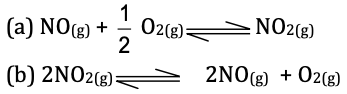Are related as:

1. a. K1 = √K2
2. b. K2 = 1 / K1
3. c. K1 = 2K2
4. d. K2 = [1 / K1,]2

Solution: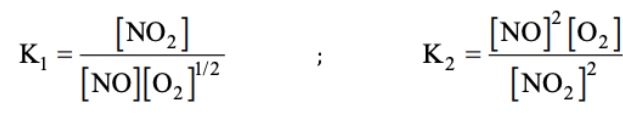If we reverse and multiply equation (a) by two then the equilibrium constant:

K2 = [1 / K1,]2

Question 30: Van-Arkel method of refining Zirconium involves,

1. a. removing all oxygen and nitrogen impurities
2. b. removing CO impurity
3. c. removing Hydrogen impurity
4. d. removing silica impurity

Solution:

Van – Arkel method (vapour phase refining).

By this method, we obtain ultrapure metal from crude metal. In this method impurities as oxygen and nitrogen present in zirconium or titanium are removed by thermal decomposition of metal.

The metal once converted into unstable metal iodides which are unstable and easily decomposes to form pure metals.

Question 31: The composition of ‘copper matte’ is,

1. a. Cu2S + FeS
2. b. Cu2S + Cu2O
3. c. Cu2S + FeO
4. d. Cu2O + FeS

Solution:

Copper matte is a mixture of copper sulphide (Cu2S) and some iron sulphide (FeS). Before the final reduction process, copper is extracted from matte. When a hot blast of air is blown through a molten matte placed in a silica lined converter, FeS of the matte oxidized to FeO.

Question 32: The complex formed when Al2O3 is leached from Bauxite using concentrated NaOH solution is,

1. a. Na[Al(OH)4]
2. b. NaAl2O4
3. c. Na2[Al(OH)3]
4. d. Na2AlO2

Solution:

Leaching: It is a chemical method of concentration. In this process, metallic ore dissolves in a suitable reagent in which metallic ore is soluble and impurities are insoluble.

Leaching of alumina from bauxite.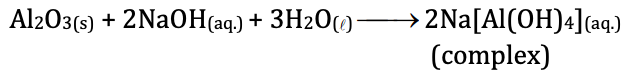Question 33: The property which is not true about Fluorine is,

1. a. Most of its reactions are exothermic.
2. b. It forms only one oxo acid.
3. c. Highest electronegativity
4. d. High F-F bond dissociation enthalpy.

Solution:

Bond dissociation enthalpy depends on bond strength. Bond strength depends on the attractive and repulsive force present in a molecule. The bond dissociation energy of F – F is minimum as the repulsion between the bond pair and lone pairs of F is strong.

Question 34: Which is true regarding nitrogen?

1. a. Less electronegative
2. b. Has low ionization enthalpy
3. c. d-orbitals are available
4. d. Ability to form pπ - pπ bonds with itself

Solution:

Nitrogen can form Pπ - Pπ bond with itself due to the small size of N-atom. Its valence electrons are close enough to form a bond with another N-atom.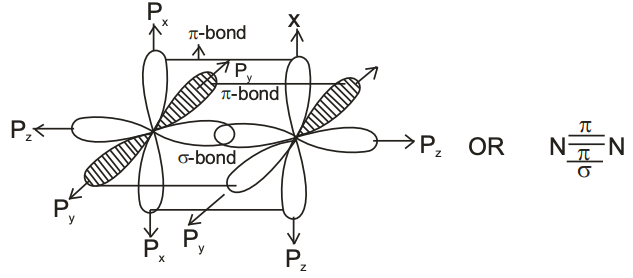Question 35: The shape of XeF6 is,

1. a. Square planar
2. b. Distorted octahedral
3. c. Square pyramidal
4. d. Pyramidal

Solution:

XeF6 molecule is Sp3d3 hybridized. After hybridization, XeF6 molecular geometry will be distorted octahedral or square bipyramidal due to the lone pair of electron.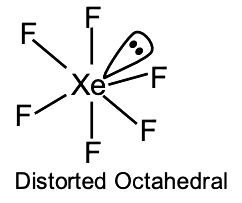Question 36: The number of isomers possible for the octahedral complex [CoCl2(en) (NH3)2]+ is,

1. a. Two
2. b. Three
3. c. No isomer
4. d. Four isomers

Solution:

Octahedral complex [CoCl2(en) (NH3)2]+

For this complex, there are two geometrical isomers possible i.e. cis and trans isomer.

Cis isomer has two optical isomers i.e. d-cis isomer and l-cis isomer.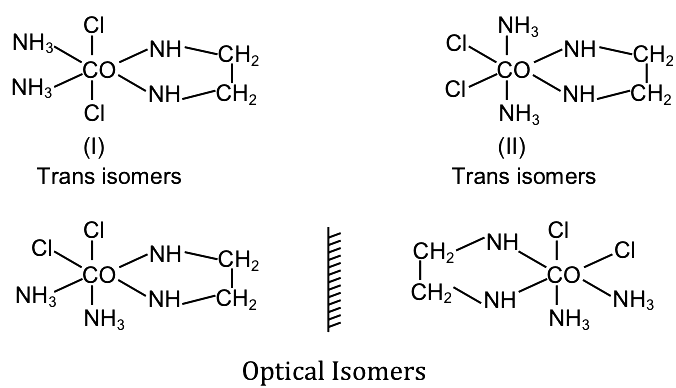Cis isomer shows optical isomerism due to absence of plane of symmetry.

Question 37: CO is a stronger ligand than Cl, because

1. a. CO is a neutral molecule.
2. b. CO has π - bonds
3. c. CO is poisonous.
4. d. CO is more reactive

Solution:

The lower HOMO energy means that Co is a poorer 𝜎 donor orbital towards the metal than Cl. Likewise, the lower LUMO makes it a better π acceptor.

These two factors are conflicting stronger π acceptor are stronger field ligands. But poorer 𝜎 donors are weaker field ligands.

Question 38: The bivalent metal ion having maximum paramagnetic behaviour among the first transition series elements is,

1. a. Mn2+
2. b. Cu2+
3. c. Sc2+
4. d. Cu+

Solution:

The paramagnetic character of the metal ion depends on the number of unpaired electrons. Maximum the number of unpaired electrons maximum is the paramagnetic behaviour of the element.

Electronic configuration:

Mn+2: [Ar] 3d5 45º n = 5

Cu+2: [Ar] 3d9 45º n = 1 {n = number of unpaired electrons}

Sc+2: [Ar] 3d1 45º n = 1

Cu+: [Ar] 3d10 451 n = 1

Among these transition metals, Mn+2 has five unpaired electrons. Having maximum paramagnetic character.

Question 39: When a brown compound of Mn (A) is treated with HCl, it gives a gas (B). The gas (B) taken in excess reacts with NH3 to give an explosive compound(C). The compounds A, B and C are;

1. a. A = MnO2, B = Cl2, C = NCl3
2. b. A = MnO, B = Cl2, C = NH4Cl
3. c. A = Mn3O4, B = Cl2, C = NCl3
4. d. A = MnO3, B = Cl2, C = NCl2

Solution:

Brown compound of manganese is MnO2 (A) treated with HCl give chlorine gas as a byproduct.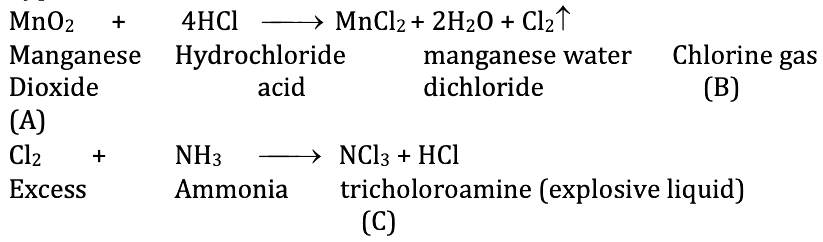So, the compound A = MnO2, B = Cl2, C = NCl3

Question 40: Mn2+ compounds are more stable than Fe2+ compounds towards oxidation their + 3 state, because

1. a. Mn2+ is more stable with high 3rd Ionisation energy
2. b. Mn2+ is bigger in size
3. c. Mn2+ has completely filled d-orbitals
4. d. Mn2+ does not exist

Solution:

1. Answer: Mn+2 outermost electronic configurations are 3d5 4s°. So, Mn+2 gain extra stability due to half field subshells. Removal of one more electron decreases the stability of this compound that is why it has higher 3rd ionization energy.

While Fe+2 has 3d6 4s° electronic configuration by losing of one more electron from d-orbital, it acquires stable half-filled configuration i.e. 3d5 4s°.

So, Mn+2 stable with half-filled configuration rather than Mn+3 and Fe+3 more stable than the Fe+2 ion.

Question 41: Which of the following sequence is correct regarding field strength of ligands as per spectrochemical series?

1. a. SCN< F < CN < CO
2. b. F < SCN < CN < CO
3. c. CN < F < CO < SCN
4. d. SCN < CO < F < CN

Solution:

A spectrochemical series is a series of ligands ordered on ligand Strength. Ligand arranged on the left end of this spectrochemical series are generally regarded as weaker ligands and cannot cause forcible pairing of electrons within the 3d level. On the other hand, stronger ligand lies at the right end and form inner orbital after forcible pairing of electrons hence, are called low spin ligands. The correct order of field strength of ligand as per spectrochemical series.

SCN< F < CN < CO

Question 42: As per IUPAC norms, the name of the complex [Co(en)2 (ONO)Cl]Cl is

1. a. Chlorido bis(ethane-1, 2-diamine) nitro-o-cobalt(III) chloride
2. b. Chloro bis(ethylene diamine) nitro-o-cobalt(III) chloride.
3. c. Chlorido di(ethylenediamine) nitro cobalt (III) chloride.
4. d. Chloro ethylene diamine nitro-o-cobalt (III) chloride.

Solution:

[Co(en)2 (ONO)Cl]Cl

Oxidation state of Co. x + 2(0) + (–1) + (–1) = +1

X = + 1 + 1 + 1 = +3

First, we write the cationic part and then anionic part. Then, we place ligands according to their alphabetical order. For bidentate ligand, we use prefix bis. After that, place metal and oxidation state of metal atom in roman number and anionic part. IUPAC name of [Co (en)2(ONO)Cl] is chlorobis (ethylenediamine) nitrito-o-cobalt (III) chloride.

Question 43: In the following sequence of reactions ;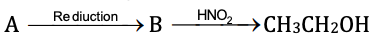The compound A is

1. a. Propane nitrile
2. b. Ethane nitrile
3. c. CH3NO2
4. d. CH3NC

Solution:

Reduction of ethane nitrile gives ethyl amine overall CN triple bond is reduced to give a primary amine. Primary amine contains the – NH2 group.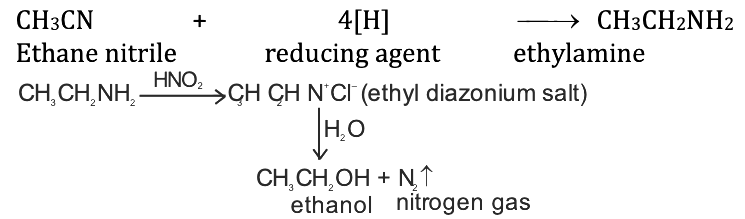Question 44: An organic compound A on reduction gives compound B, which on reaction with trichloromethane and caustic potash forms C. The compound ‘C’ on catalytic reduction gives N-methyl benzenamine, the compound ‘A’ is,

1. a. Nitrobenzene
2. b. Nitromethane
3. c. Methanamine
4. d. Benzenamine

Solution: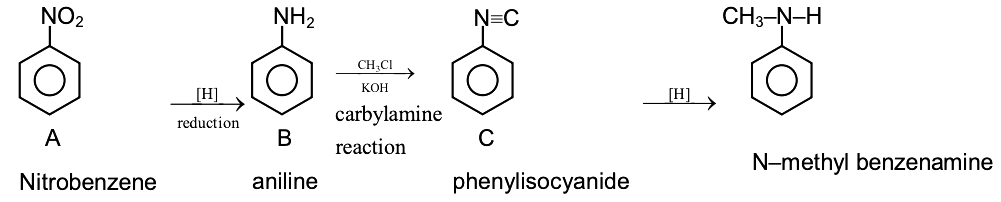In carbylamines reaction 1° amine reacts with trichloromethane and caustic potash (KOH) to form isocyanide compound. On behalf of 1º amine, we can take aniline as a reduction product of nitrobenzene. So the compound A is Nitrobenzene.

Question 45: Which of the following gives positive Fehling’s solution test?

1. a. Sucrose
2. b. Glucose
3. c. Fats
4. d. Protein

Solution:

Fehling’s solution is a chemical reagent used to differentiate between water-soluble carbohydrate and ketone functional groups and as a test for reducing sugar and non reducing sugar, supplementary to the tollen’s reagent test. Glucose is a reducing sugar and contains aldehyde group to give a positive test with Fehling’s solution. Sucrose is non reducing sugar does not give a test.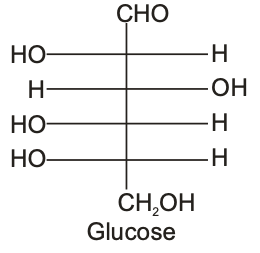Question 46: A liquid can exist only,

1. a. Between triple point and critical point
2. b. At any temperature above melting point
3. c. Between melting point and critical point
4. d. Between boiling and melting points.

Solution:

The critical point is the highest temperature and pressure at which a pure material can exist in vapour/liquid equilibrium. At a temperature higher than the critical temperature, the substance cannot exist as a liquid, no matter what the pressure. Since the triple point is a point there is only one temperature and one pressure where the three phases will exist.

Question 47: The energy of an electron in the nth Bohr orbit of H-atom is

1. a. [-13.6 / n2] eV
2. b. [-13.6 / n] eV
3. c. [-13.6 / n4] eV
4. d. [-13.6 / n3] eV

Solution:

Energy of electron in nth Bohr - orbit of H-atom

Total energy K.E + P.E.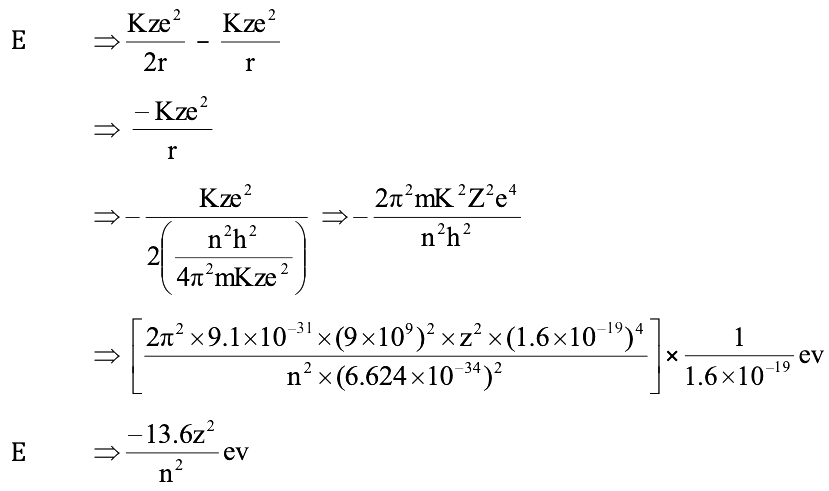Question 48: Consider the following sets of quantum numbers: Which of the below setting is not a permissible arrangement of electrons in an atom?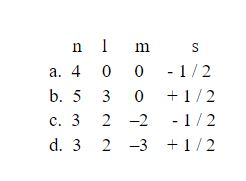Solution:

The magnetic Quantum number (ml) determines the number of orbitals and their orientation. Consequently, its value depends on the orbital angular quantum number (l).

If n = 3, l = 2, m = –3, s = + 1 / 2

For this arrangement, m must be in the range from – l to +l,

Then ml can vary from –2, –1, 0, +1, +2

Hence the option (4) is incorrect.

Question 49: The increasing order of bond order O2, O2+, O2 and O2−− is

1. a. O2+, O2, O2 and O2−−
2. b. O2−−, O2, O2+, and O2
3. c. O2, O2+, O2 and O2−−
4. d. O22-, O2-, O2 and O2+

Solution:

Bond order formula Bond order = (1 / 2) × [number of an electron in anti-bonding molecular orbitals – [number of electrons in bonding molecular orbitals]

(i) B.O of O2 = (1 / 2) × [10 – 6] = 2

(ii) B.O of O2+ = (1 / 2) × [10 – 5] = 2.5

(iii) B.O of O2– = (1 / 2) × [10 – 7] = 1.5

(iv) B.O of O2^–2 = (1 / 2) × [10 – 8] = 1

Increasing bond order is: O22-, O2-, O2 and O2+

Question 50: HCl gas is covalent and NaCl is an ionic compound. This is because

1. a. Sodium is highly electro +Ve
2. b. Hydrogen is a non-metal.
3. c. HCl is a gas
4. d. The electronegativity difference between H and Cl is less than 2.1.

Solution:

The electronegativity difference between atoms helps to determine the compound’s ionic or covalent character. If the Electronegativity is large, the more negative and positive the atoms became.

NaCl (sodium chloride) is ionically bonded. Because Sodium has E.N of 1.0 and chlorine has an E.N of 3.0

ΔEN = 3.0 – 1.0 = 2.0

The large difference in Electronegativity, make the bond between the two atoms highly polar.

In Hydrogen chloride(HCl). Hydrogen has an Electronegativity of 2.1 and chlorine has 3.0. The electron pair that is bonding HCl together, shifts towards the chlorine atom because it has a large Electronegativity value. So, HCl gas is covalent.

Question 51: Which of the following is not true?

1. a. In vulcanization, the rubber becomes harder and stronger.
2. b. Natural rubber has ‘trans’ configuration at every double bond.
3. c. Buna-S is a co-polymer of Butene and styrene.
4. d. Natural rubber is 1, 4-polymer of isoprene.

Solution:

Natural rubber is a linear polymer of isoprene called as cis –1,4-polyisoprene.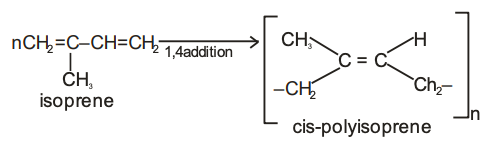Buna –S: It is also known as styrene-butadiene rubber (SBR). It is obtained by the polymerization of butadiene and styrene in the ratio of 3:1 in presence of sodium.

Question 52: Which of the following is a polyamide?

1. a. Nylon-6, 6
2. b. Terylene
3. c. Polythene
4. d. Buna-S

Solution:

→ Polyamides are the polycondensation product of a diacid chloride and a diamine Nylon-6,6 is polyamide polymer with repeating units linked by amide bonds.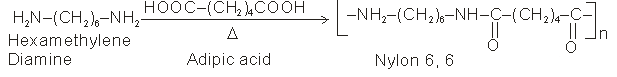→ Terylene or Dacron is a polyester polymer that contains the ester functional group in its main chain. Glycol + Terepthalic acid → Terylene

→ Buna-S is synthetic rubber polymer.

Question 53: Which of the following is correct about H-bonding in DNA?

1. a. A – T, G – C
2. b. A – G, T – C
3. c. G – T, A – C
4. d. A – A, T – T

Solution:

DNA is a double helix structure in which two nucleic acid chains are wound about each other and hold together by a Hydrogen bond between pairs of bases. Adenine forms a Hydrogen bond with thymine whereas cytosine Hydrogen bonds with guanine.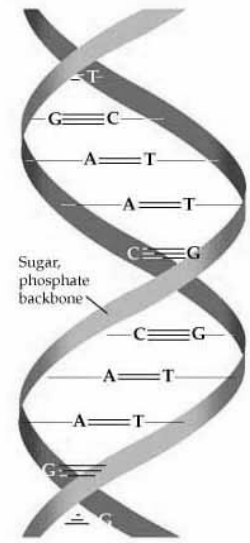Question 54: Which of the following is employed as Tranquilizer?

1. a. Equanil
2. b. Naproxen
3. c. Tetracyclin
4. d. Dettol

Solution:

Tranquillizers (antidepressant drugs):

The chemical which is used to reduce mental tension relieves anxiety and mental stress.

i.e. Equanil

• Anti-inflammatory drugs used to treat such as headache, muscle aches etc. i.e Naproxen.
• Tetracycline is an important class of antibiotics. It is used as a treatment of typhoid and treatment of eyes.
• Dettol is commonly used antiseptic, the chemical which either kills or prevents the growth of microorganisms.

Question 55: Reactivity of order of halides for dehydrohalogenation is

1. a. R – F > R – Cl> R – Br > R – I
2. b. R – I > R – Br > R – Cl> R – F
3. c. R – I > R – Cl> R – Br > R – F
4. d. R – F > R – l > R – Br > R – Cl

Solution:

F has the smallest size among the group. I has the largest size. As the size of the atom increases bond length also increases which makes bond easily to break. Bond length order of halides:

R –I > R – Br > R – Cl > R – F

As the bond length becomes large, the compound becomes less stable readily breaks. Reactivity order of dehydrohalogenation of alkyl halides:

R – I > R – Br > R – Cl> R – F

Question 56: The main axis of the diatomic molecule is Z. The orbitals Px and Py overlap to form

1. a. π-molecular orbital
2. b. σ-molecular orbital
3. c. δ-molecular orbital
4. d. No bond is formed

Solution:

Z-axis is the internuclear axis. There is no effective overlapping in labs. So, No bond formation between these labs.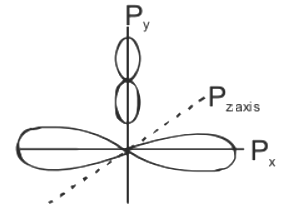Question 57: The hybridisation of C in diamond, graphite and ethyne is in the order

1. a. sp3, sp, sp2
2. b. sp3, sp2, sp
3. c. sp, sp2, sp3
4. d. sp2, sp3, sp

Solution:

Hybridization of C in diamond:-

The electronic configuration of carbon is 1S2, 2S2 2P2, i.e. with four valence electrons spread in the S and P orbitals. In order to create a covalent bond in diamond, the S orbital mixes with the three P orbitals to form SP3 hybridisation.

Hybridization of graphite:-

Graphite has a SP2 type of hybridization. Carbon has four outermost valance electrons spread in S and P orbitals. During hybridization, the S-orbital with two P-orbitals to Additionally, each carbon atom will also consist of one non-bonded outer electrons, which becomes delocalized.

Hybridization of ethyne:-

The carbon atom consists of four outermost electrons and hydrogen has only one.

Ground state 6C: 1S2, 2S2 2Px1 2Py1 2Pz

Exited state 6C: 1S2, 2S2, 2Px1, 2Py1, Pz

So, here one S and two P orbitals participate in hybridization. The hybridization of ethyne is SP. The hybridization of C in diamond, Graphite and Ethyne is in the order: SP3, SP2, SP.

Question 58: A miscible mixture of C6H6 + CHCl3 can be separated by

1. a. Sublimation
2. b. Distillation
3. c. Filtration
4. d. Crystallisation

Solution:

Distillation process refers to the selective boiling and subsequent condensation of a component in a liquid mixture. A miscible mixture of C6H6 + CHCl3 can be separated by a simple distillation method according to their boiling points. The boiling point of chloroform is (61.2 °C) is lower than the boiling point of benzene (80.1 °C). Chloroform short boil first, vaporize and collect before benzene starts vaporize.

Question 59: An organic compound contains C = 40%, H = 13.33 % and N = 46.67 %. Its empirical formula is

1. a. C2H2N
2. b. C3H7N
3. c. CH4N
4. d. CHN

Solution:

Given that

% of C = 40%

% of H = 13.33%

% of N = 46.61%

Assume a 100g sample, convert the same % values to grams

40g carbon

13.33g Hydrogen

46.67g Nitrogen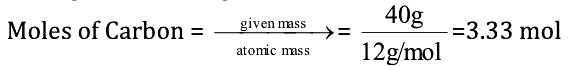Moles of Hydrogen = 13.33g / [1g/mol] = 13.33 mol

Moles of Nitrogen = 46.67g / [14g/mol] = 3.33 mol

After dividing the smallest resultant mole by each atom

C = 3.33 / 3.33 = 1; H = 3.33 / 13.33 = 4; N = 3.33 / 3.33 = 1

The formula of the compound is C1H4N1 or CH4N.

Question 60: Electrophile that participates in nitration of benzene is

1. a. NO+
2. b. NO2+
3. c. NO
4. d. NO3-

Solution:

Nitration of Benzene:

Benzene reacts with concentrated nitric acid in the presence of concentrated sulphuric acid to form nitrobenzene.

Mechanism: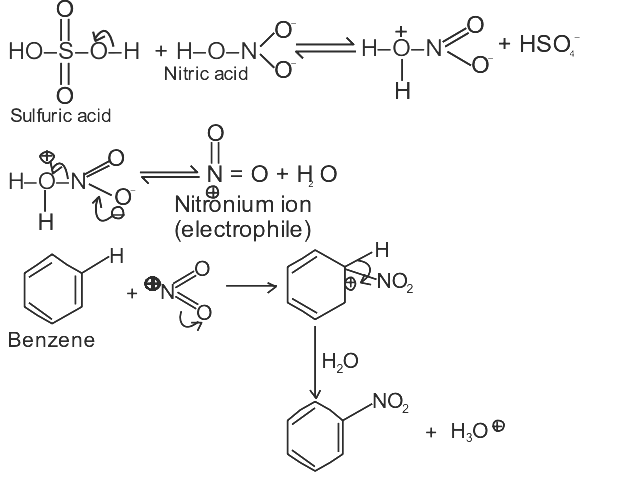### KCET 2016 Chemistry Question Paper with Solutions